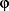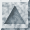9. THE CALCULATION OF THE STEREO BASE

As was stated in chapter 4, "The Exposure Base", the near-point/far-point separation difference, in short: "deviation", is not a constant, but rather a professional agreement. If, however, one agrees to use the 1/30-rule and the value derived from it for= 1.2 mm for miniature formats and approx. 3 mm for a format of 2x 6x6 (6x13), as one has for amateur stereo photography, it must be held constant within the equations. If one wishes to use other values, i.e., for photogrammetry, the graphs 1 - 3 should not be used, but should instead be taken from the ladder table, graph 6 (see appendix).

Prerequisite:
Deviation = constant
bN - bF= constant

For simplifications, assume:

bF = bO

The triangle with the opposite anglesare similar, therefore:

 bO= (= tg) 2 * aN 2 a' 1 1 bO * =* aN a' 1 1 1 1 1 1 = - ; bO * =* ( - ) a' f a aN f a 1 1 bO =* aN * ( - ) f a aN aN bO =* ( - ) f a aN aN assume = 1 ; bO =* ( - 1) a f

Since the value of a', the image distance in the camera, can hardly be measured, it is replaced with help of a well-known optical formula.
The assumption: aN/a = 1 distorts the result only ever so slightly. With a large amount of depth of field ts, a can be significantly larger when aN is big.

The value aN/f then is very large. With a small aN the value of a, the object distance = focal range, is not much larger, since then the depth of field ts, dependent on the aperture, is also not large.

The value of aN/f is then small too. Subtracting 1 or 0.8, for example, makes no difference.

According to the above equation, the stereo base bO is dependent upon the selected stereo window distance from whichis derived, from the focal length f and the near-point distance aNand usually f are constants so that only the near-point distance remains. The far-point distance is not of importance and must be considered in the choice of the aperture.

If one calculates the enlargement factor V from the known formulas for the focal length f, object- and image distance a, a' and substitutes them into the equation for the calculation of the base, this equation simplifies to bs/V.

Since both the depth of field and the exposure factor are dependent on the enlargement factor, all the relations can be depicted on graph 4. This is of special interest to macro- and micro-stereo-photography, where a lot of work involves the enlargement factor. Graph 5 shows the base dependent on the enlargement factor with different focal lengths = picture diagonals.Please do not forget to visit the Stereoscopy.com Bookshop, offering the world's largest selection of books in 3D and about 3D.Back to the Services Page